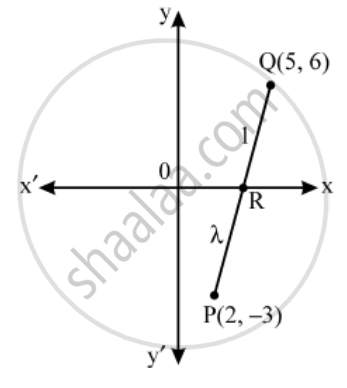# In what ratio does the x-axis divide the line segment joining the points (2, –3) and (5, 6)? Also, find the coordinates of the point of intersection. - Mathematics

Sum

In what ratio does the x-axis divide the line segment joining the points (2, –3) and (5, 6)? Also, find the coordinates of the point of intersection.

#### Solution

Let the required ratio be λ : 1. Then, the coordinates of the point of division are,

R( \frac{5\lambda +2}{\lambda +1},\ \frac{6\lambda -3}{\lambda +1})But, it is a point on x-axis on which y-coordinates of every point is zero.

\therefore \frac{6\lambda -3}{\lambda +1}=0

\Rightarrow \lambda =\frac{1}{2}

Thus, the required ratio is 1/2 : 1 or 1 : 2

Concept: Section Formula
Is there an error in this question or solution?

Share